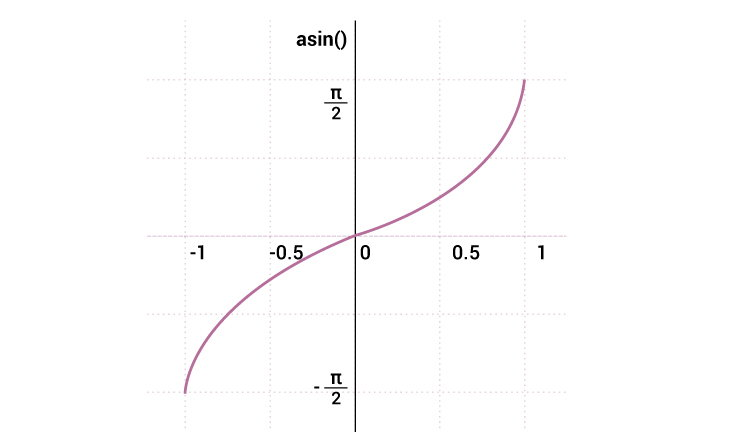# C asin()

The asin() function returns the arc sine (inverse sine) of a number in radians.The `asin()` function takes a single argument (1 ≥ x ≥ -1), and returns the arc sine in radians.

The `asin()` function is included in `<math.h>` header file.

### asin() Prototype

`double asin(double x);`

To find arc sine of type `int`, `float` or `long double`, you can explicitly convert the type to `double` using cast operator.

``` int x = 0;
double result;
result = asin(double(x));```

Also, two functions asinf() and asinl() were introduced in C99 to work specifically with type `float` and `long double` respectively.

```float asinf(float x);
long double asinl(long double x);```

### asin() Parameter

The `asin()` function takes a single argument in the range of [-1, +1]. It's because the value of sine is in the range of 1 and -1.

Parameter Description
double value Required. A double value between - 1 and +1 inclusive.

### asin() Return Value

The `asin()` functions returns the value in range of [-π/2, +π/2] in radians. If the parameter passed to the `asin()` function is less than -1 or greater than 1, the function returns NaN (not a number).

Parameter (x) Return Value
x = [-1, +1] [-π/2, +π/2] in radians
-1 > x or x > 1 NaN (not a number)

## Example 1: asin() function with different parameters

``````#include <stdio.h>
#include <math.h>
int main()
{
// constant PI is defined
const double PI =  3.1415926;
double x, result;

x =  -0.5;
result = asin(x);
printf("Inverse of sin(%.2f) = %.2lf in radians\n", x, result);

result = asin(x)*180/PI;
printf("Inverse of sin(%.2f) = %.2lf in degrees\n", x, result);

// paramter not in range
x = 1.2;
result = asin(x);
printf("Inverse of sin(%.2f) = %.2lf", x, result);

return 0;
}
``````

Output

```Inverse of sin(-0.50) = -0.52 in radians
Inverse of sin(-0.50) = -30.00 in degrees
Inverse of sin(1.20) = nan
```

## Example 2: asinf() and asinl() function

``````#include <stdio.h>
#include <math.h>
int main()
{
float fx, fasinx;
long double lx, ldasinx;

// arc sinine of type float
fx = -0.505405;
fasinx = asinf(fx);

// arc sinine of type long double
lx = -0.50540593;
ldasinx = asinf(lx);

printf("asinf(x) = %f in radians\n", fasinx);
printf("asinl(x) = %Lf in radians", ldasinx);

return 0;
}``````

Output

```asinf(x) = -0.529851 in radians